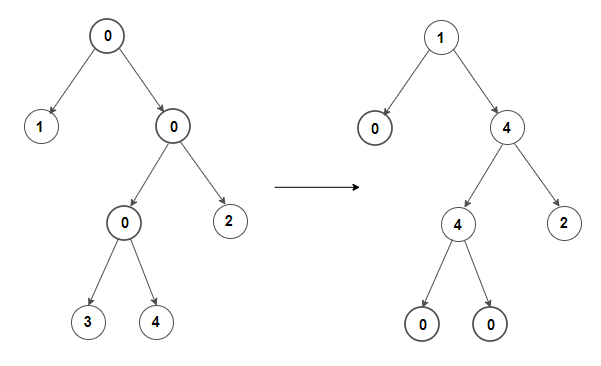# Sink nodes containing zero to the bottom of the binary tree

Given a binary tree containing many zero nodes, sink nodes having zero value to the bottom of the sub-tree rooted at that node. In other words, the output binary tree should not contain any node having zero value that is parent of node having non-zero value.

For example, binary tree shown on the left can be converted to binary tree shown on the rightThe idea is to process the tree nodes in postorder manner, i.e. after fixing left and right subtrees of a node, we fix the node if it is zero. To fix a node, we do something similar to Heapify procedure of Heap sort. As left and right subtree are already fixed, we can fix the binary tree rooted at current node by moving the current node (containing zero) down the tree. We do so by comparing the node with its left and right child and swapping it with the non-zero child and then recursively calling the procedure on the corresponding child. The process is repeated till leaf node is reached or subtree rooted at current node contains all zeroes. As several binary trees can be constructed from one input, below solution would construct any one of them.

C++ implementation –

The time complexity of above solution is O(n2) and need O(h) extra space for the call stack where h is the height of the tree.(1 votes, average: 5.00 out of 5)Loading...

Please use our online compiler to post code in comments. To contribute, get in touch with us.
Like us? Please spread the word and help us grow. Happy coding 🙂